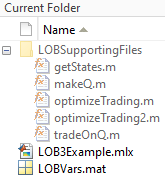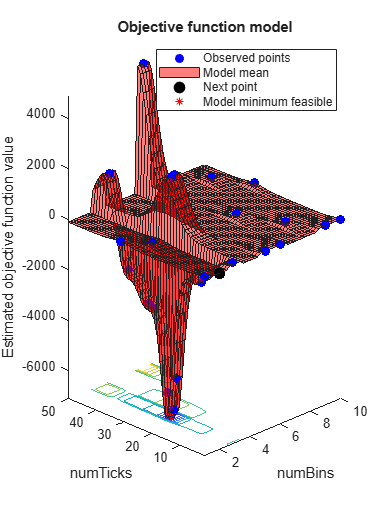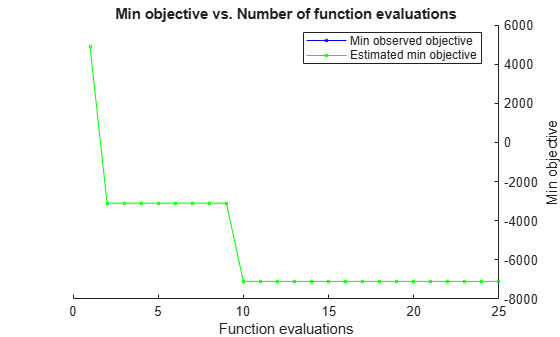# Machine Learning for Statistical Arbitrage III: Training, Tuning, and Prediction

This example uses Bayesian optimization to tune hyperparameters in the algorithmic trading model, supervised by the end-of-day return. It is part of a series of related examples on machine learning for statistical arbitrage (see Machine Learning Applications).

Load `LOBVars.mat`, the preprocessed LOB data set of the NASDAQ security INTC.

`load LOBVars`

The data set contains the following information for each order: the arrival time `t` (seconds from midnight), level 1 asking price `MOAsk`, level 1 bidding price `MOBid`, midprice `S`, and imbalance index `I`.

This example includes several supporting functions, which are stored in `matlabroot/examples/finance/data/LOBSupportingFiles`. To view them, change your working folder.

`cd(fullfile(matlabroot,'examples','finance','data','LOBSupportingFiles'));`

The trading matrix `Q` contains probabilities of future price movements, given current and previous states `rho` of the limit order book (LOB) imbalance index `I` and the latest observed direction in prices `DS`.

View the supporting function `tradeOnQ.m`, which implements a simple trading strategy based on the pattern in `Q`.

```function cash = tradeOnQ(Data,Q,n,N) % Reference: Machine Learning for Statistical Arbitrage % Part II: Feature Engineering and Model Development % Data t = Data.t; MOBid = Data.MOBid; MOAsk = Data.MOAsk; % States [rho,DS] = getStates(Data,n,N); % Start of trading cash = 0; assets = 0; % Active trading T = length(t); for tt = 2:T-N % Trading ticks % Get Q row, column indices of current state row = rho(tt-1)+n*(DS(tt-1)+1); downColumn = rho(tt); upColumn = rho(tt) + 2*n; % If predicting downward price move if Q(row,downColumn) > 0.5 cash = cash + MOBid(tt); % Sell assets = assets - 1; % If predicting upward price move elseif Q(row,upColumn) > 0.5 cash = cash - MOAsk(tt); % Buy assets = assets + 1; end end % End of trading (liquidate position) if assets > 0 cash = cash + assets*MOBid(T); % Sell off elseif assets < 0 cash = cash + assets*MOAsk(T); % Buy back end ```

The algorithm uses predictions from `Q` to make decisions about trading at each tick. It illustrates the general mechanism of any optimized machine learning algorithm.

This strategy seeks to profit from expected price changes using market orders (best offer at the touch) of a single share at each tick, if an arbitrage opportunity arises. The strategy can be scaled up to larger trading volumes. Using the conditional probabilities obtained from `Q`, the `tradeOnQ` function takes one of these actions:

• Executes a buy if the probability of an upward forward price change is greater than 0.5.

• Executes a sell if the probability of an downward forward price change is greater than 0.5.

At the end of the trading day, the function liquidates the position at the touch.

The strategy requires `Data` with tick times `t` and the corresponding market order bid and ask prices `MOBid` and `MOAsk`, respectively. In real-time trading, data is provided by the exchange. This example evaluates the strategy by dividing the historical sample into training (calibration) and validation subsamples. The validation subsample serves as a proxy for real-time trading data. The strategy depends on `Q`, the trading matrix itself, which you estimate after you make a number of hyperparameter choices. The inputs `n` and `N` are hyperparameters to tune when you optimize the strategy.

### Hyperparameters

The continuous-time Markov model and the resulting trading matrix `Q` depend on the values of four hyperparameters:

• `lambda` — The weighting parameter used to compute the imbalance index `I`

• `dI` — The number of backward ticks used to average `I` during smoothing

• `numBins` — The number of bins used to partition smoothed `I` for discretization

• `dS` — The number of forward ticks used to convert the prices `S` to discrete `DS`

In general, all four hyperparameters are tunable. However, to facilitate visualization, the example reduces the number of dimensions by tuning only `numBins` and `N`. The example:

• Fixes `lambda`

• Leaves `numBins` = `n`, where `n` is free to vary

• Equalizes the window lengths `dI` = `dS` = `N`, where `N` is free to vary

The restrictions do not significantly affect optimization outcomes. The optimization algorithm searches over the two-dimensional parameter space (`n`,`N`) for the configuration yielding the maximum return on trading.

### Training and Validation Data

Machine learning requires a subsample on which to estimate `Q` and another subsample on which to evaluate the hyperparameter selections.

Specify a breakpoint to separate the data into training and validation subsamples. The breakpoint affects evaluation of the objective function, and is essentially another hyperparameter. However, because you do not tune the breakpoint, it is external to the optimization process.

`bp = round((0.80)*length(t)); % Use 80% of data for training`

Collect data in a timetable to pass to `tradeOnQ`.

```Data = timetable(t,S,I,MOBid,MOAsk); TData = Data(1:bp,:); % Training data VData = Data(bp+1:end,:); % Validation data```

### Cross-Validation

Cross-validation describes a variety of techniques to assess how training results (here, computation of `Q`) generalize, with predictive reliability, to independent validation data (here, profitable trading). The goal of cross-validation is to flag problems in training results, like bias and overfitting. In the context of the trading strategy, overfitting refers to the time dependence, or nonstationarity, of `Q`. As `Q` changes over time, it becomes less effective in predicting future price movements. The key diagnostic issue is the degree to which `Q` changes, and at what rate, over a limited trading horizon.

With training and validation data in place, specify the hyperparameters and compare `Q` in the two subsamples. The supporting function `makeQ.m` provides the steps for making `Q`.

```% Set specific hyperparameters n = 3; % Number of bins for I N = 20; % Window lengths % Compare Qs QT = makeQ(TData,n,N); QV = makeQ(VData,n,N); QTVDiff = QT - QV```
```QTVDiff = 9×9 0.0070 0.0182 0.1198 -0.0103 -0.0175 -0.0348 0.0034 -0.0007 -0.0851 -0.0009 0.0176 0.2535 -0.0010 -0.0233 -0.2430 0.0019 0.0058 -0.0106 0.0184 0.0948 0.0835 -0.0195 -0.1021 -0.1004 0.0011 0.0073 0.0168 0.0462 0.0180 0.0254 -0.0512 -0.0172 0.0417 0.0050 -0.0009 -0.0671 0.0543 0.0089 0.0219 -0.0556 -0.0169 -0.0331 0.0013 0.0080 0.0112 0.1037 0.0221 0.0184 -0.1043 -0.0401 -0.0479 0.0006 0.0180 0.0295 0.0266 0.0066 0.0054 -0.0821 -0.0143 -0.0116 0.0555 0.0077 0.0062 0.0615 0.0050 0.0060 -0.0189 -0.0207 -0.0262 -0.0426 0.0157 0.0203 0.0735 0.0103 0.0090 -0.0788 -0.1216 -0.0453 0.0053 0.1113 0.0362 ```

Differences between `QT` and `QV` appear minor, although they vary based on their position in the matrix. Identify trading inefficiencies, which result from indices (market states) where one matrix gives a trading cue (probability value > 0.5) and the other does not.

`Inhomogeneity = (QT > 0.5 & QV < 0.5 ) | (QT < 0.5 & QV > 0.5 )`
```Inhomogeneity = 9x9 logical array 0 0 0 0 0 0 0 0 0 0 0 0 0 0 0 0 0 0 0 0 0 0 0 0 0 0 0 0 0 0 0 0 0 0 0 0 0 0 0 0 0 0 0 0 0 0 0 0 0 0 0 0 0 0 0 0 0 0 0 0 0 0 0 0 0 0 0 0 0 0 0 0 0 0 0 0 0 0 0 0 0 ```

No significant inhomogeneities appear in the data with the given hyperparameter settings.

The severity of proceeding with a homogeneity assumption is not known a priori, and can emerge only from more comprehensive backtesting. Statistical tests are available, as described in  and , for example. During real-time trading, a rolling computation of `Q` over trailing training data of suitable size can provide the most reliable cues. Such an approach acknowledges inherent nonstationarity in the market.

### Machine Learning

Machine learning refers to the general approach of effectively performing a task (for example, trading) in an automated fashion by detecting patterns (for example, computing `Q`) and making inferences based on available data. Often, data is dynamic and big enough to require specialized computational techniques. The evaluation process—tuning hyperparameters to describe the data and direct performance of the task—is ongoing.

In addition to the challenges of working with big data, the process of evaluating complex, sometimes black-box, objective functions is also challenging. Objective functions supervise hyperparameter evaluation. The trading strategy evaluates hyperparameter tunings by first computing `Q` on a training subsample, and then trading during an evaluation (real-time) subsample. The objective is to maximize profit, or minimize negative cash returned, over a space of suitably constrained configurations (`n`,`N`). This objective is a prototypical "expensive" objective function. Bayesian optimization is a type of machine learning suited to such objective functions. One of its principle advantages is the absence of costly derivative evaluations. To implement Bayesian optimization, use the Statistics and Machine Learning Toolbox™ function `bayesopt`.

The supporting function `optimizeTrading.m` uses `bayesopt` to optimize the trading strategy in `tradeOnQ`.

```function results = optimizeTrading(TData,VData) % Optimization variables n = optimizableVariable('numBins',[1 10],'Type','integer'); N = optimizableVariable('numTicks',[1 50],'Type','integer'); % Objective function handle f = @(x)negativeCash(x,TData,VData); % Optimize results = bayesopt(f,[n,N],... 'IsObjectiveDeterministic',true,... 'AcquisitionFunctionName','expected-improvement-plus',... 'MaxObjectiveEvaluations',25,... 'ExplorationRatio',2,... 'Verbose',0); end % optimizeTrading % Objective (local) function loss = negativeCash(x,TData,VData) n = x.numBins; N = x.numTicks; % Make trading matrix Q Q = makeQ(TData,n,N); % Trade on Q cash = tradeOnQ(VData,Q,n,N); % Objective value loss = -cash; end % negativeCash ```

Optimize the trading strategy by passing the training and validation data to `optimizeTrading`.

```rng(0) % For reproducibility results = optimizeTrading(TData,VData); ```The estimated minimum objective coincides with the minimum observed objective (the search is monotonic). Unlike derivative-based algorithms, `bayesopt` does not converge. As it tries to find the global minimum, `bayesopt` continues exploring until it reaches the specified number of iterations (`25`).

Obtain the best configuration by passing the `results` to `bestPoint`.

`[Calibration,negReturn] = bestPoint(results,'Criterion','min-observed')`
```Calibration=1×2 table numBins numTicks _______ ________ 3 24 ```
```negReturn = -7100 ```

Trading one share per tick, as directed by `Q`, the optimal strategy using (`n`,`N`) = (3,24) returns \$0.71 over the final 20% of the trading day. Modifying the trading volume scales the return.

Another optimizer designed for expensive objectives is `surrogateopt` (Global Optimization Toolbox™). It uses a different search strategy and can locate optima more quickly, depending on the objective. The supporting function `optimizeTrading2.m` uses `surrogateopt` instead of `bayesopt` to optimize the trading strategy in `tradeOnQ`.

```rng(0) % For reproducibility results2 = optimizeTrading2(TData,VData)```
```Scalar objective function Number of variables: 2 Number of integer constraints: 2 Number of inequality constraints: 0 F-count Time Best Current Trial (seconds) Fval Fval type 1 1.25 -0.0000e+00 -0.0000e+00 random ```
``` 2 2.31 -0.0000e+00 -0.0000e+00 random ```
``` 3 2.74 -1.3000e+03 -1.3000e+03 random ```
``` 4 3.15 -1.3000e+03 -0.0000e+00 random ```
``` 5 3.55 -1.3000e+03 1.1000e+03 random ```
``` 6 4.20 -1.3000e+03 8.0000e+02 adaptive ```
``` 7 4.73 -1.3000e+03 -0.0000e+00 adaptive ```
``` 8 5.09 -3.1000e+03 -3.1000e+03 adaptive ```
``` 9 5.47 -4.3000e+03 -4.3000e+03 adaptive ```
``` 10 5.86 -6.8000e+03 -6.8000e+03 adaptive ```
``` 11 6.24 -6.8000e+03 -0.0000e+00 adaptive ```
``` 12 6.54 -6.8000e+03 -6.2000e+03 adaptive ```
``` 13 6.83 -7.1000e+03 -7.1000e+03 adaptive ```
``` 14 7.24 -7.1000e+03 -0.0000e+00 adaptive ```
``` 15 7.55 -7.1000e+03 -5.8000e+03 adaptive ```
``` 16 7.90 -7.1000e+03 1.1000e+03 adaptive ```
``` 17 8.30 -7.1000e+03 -5.5000e+03 adaptive ```
``` 18 8.69 -7.1000e+03 -0.0000e+00 adaptive ```
``` 19 8.96 -7.1000e+03 -0.0000e+00 adaptive ```
``` 20 9.31 -7.1000e+03 -5.3000e+03 adaptive ```
``` 21 9.59 -7.1000e+03 -4.4000e+03 adaptive ```
``` 22 9.99 -7.1000e+03 -0.0000e+00 adaptive ```
``` 23 10.30 -7.1000e+03 -0.0000e+00 adaptive ```
``` 24 10.70 -7.1000e+03 -0.0000e+00 adaptive ```
``` 25 11.02 -7.1000e+03 -4.5000e+03 adaptive ``````Surrogateopt stopped because it exceeded the function evaluation limit set by 'options.MaxFunctionEvaluations'. ```
```results2 = 1×2 3 24 ```

The results obtained with `surrogateopt` are the same as the `bayesopt` results. The plot contains information about the progress of the search that is specific to the `surrogateopt` algorithm.

Compute `Q` by passing the optimal hyperparameters and the entire data set to `makeQ`.

`bestQ = makeQ(Data,3,24)`
```bestQ = 9×9 0.3933 0.1868 0.1268 0.5887 0.7722 0.6665 0.0180 0.0410 0.2068 0.5430 0.3490 0.2716 0.4447 0.6379 0.6518 0.0123 0.0131 0.0766 0.6197 0.3897 0.3090 0.3705 0.5954 0.6363 0.0098 0.0150 0.0547 0.1509 0.0440 0.0261 0.8217 0.8960 0.6908 0.0273 0.0601 0.2831 0.1900 0.0328 0.0280 0.7862 0.9415 0.8316 0.0238 0.0257 0.1404 0.2370 0.0441 0.0329 0.7391 0.9221 0.8745 0.0239 0.0338 0.0925 0.1306 0.0234 0.0101 0.7861 0.6566 0.4168 0.0833 0.3200 0.5731 0.1276 0.0169 0.0118 0.7242 0.6505 0.4712 0.1482 0.3326 0.5171 0.1766 0.0282 0.0186 0.7216 0.7696 0.6185 0.1018 0.2023 0.3629 ```

The trading matrix `bestQ `can be used as a starting point for the next trading day.

### Summary

This example implements the optimized trading strategy developed in the first two related examples. Available data is split into training and validation subsamples and used, respectively, to compute the trading matrix `Q` and execute the resulting trading algorithm. The process is repeated over a space of hyperparameter settings using the global optimizers `bayesopt` and `surrogateopt`, both of which identify an optimal strategy yielding a positive return. The approach has many options for further customization.

 Bull, Adam D. "Convergence Rates of Efficient Global Optimization Algorithms." Journal of Machine Learning Research 12, (November 2011): 2879–904.

 Rubisov, Anton D. "Statistical Arbitrage Using Limit Order Book Imbalance." Master's thesis, University of Toronto, 2015.

 Snoek, Jasper, Hugo Larochelle, and Ryan P. Adams. "Practical Bayesian Optimization of Machine Learning Algorithms." In Advances in Neural Information Processing Systems 25, F. Pereira et. al. editors, 2012.

 Tan, Barış, and Kamil Yılmaz. “Markov Chain Test for Time Dependence and Homogeneity: An Analytical and Empirical Evaluation.” European Journal of Operational Research 137, no. 3 (March 2002): 524–43. https://doi.org/10.1016/S0377-2217(01)00081-9.

 Weißbach, Rafael, and Ronja Walter. “A Likelihood Ratio Test for Stationarity of Rating Transitions.” Journal of Econometrics 155, no. 2 (April 2010): 188–94. https://doi.org/10.1016/j.jeconom.2009.10.016.

﻿